# Discrete Mathematics - Sets

German mathematician G. Cantor introduced the concept of sets. He had defined a set as a collection of definite and distinguishable objects selected by the means of certain rules or description.

Set theory forms the basis of several other fields of study like counting theory, relations, graph theory and finite state machines. In this chapter, we will cover the different aspects of Set Theory.

## Set - Definition

A set is an unordered collection of different elements. A set can be written explicitly by listing its elements using set bracket. If the order of the elements is changed or any element of a set is repeated, it does not make any changes in the set.

### Some Example of Sets

• A set of all positive integers
• A set of all the planets in the solar system
• A set of all the states in India
• A set of all the lowercase letters of the alphabet

## Representation of a Set

Sets can be represented in two ways −

• Roster or Tabular Form
• Set Builder Notation

### Roster or Tabular Form

The set is represented by listing all the elements comprising it. The elements are enclosed within braces and separated by commas.

Example 1 − Set of vowels in English alphabet, $A = \lbrace a,e,i,o,u \rbrace$

Example 2 − Set of odd numbers less than 10, $B = \lbrace 1,3,5,7,9 \rbrace$

### Set Builder Notation

The set is defined by specifying a property that elements of the set have in common. The set is described as $A = \lbrace x : p(x) \rbrace$

Example 1 − The set $\lbrace a,e,i,o,u \rbrace$ is written as −

$A = \lbrace x : \text{x is a vowel in English alphabet} \rbrace$

Example 2 − The set $\lbrace 1,3,5,7,9 \rbrace$ is written as −

$B = \lbrace x : 1 \le x \lt 10 \ and\ (x \% 2) \ne 0 \rbrace$

If an element x is a member of any set S, it is denoted by $x \in S$ and if an element y is not a member of set S, it is denoted by $y \notin S$.

ExampleIf $S = \lbrace1, 1.2, 1.7, 2\rbrace , 1 \in S$ but $1.5 \notin S$

### Some Important Sets

N − the set of all natural numbers = $\lbrace1, 2, 3, 4, .....\rbrace$

Z − the set of all integers = $\lbrace....., -3, -2, -1, 0, 1, 2, 3, .....\rbrace$

Z+ − the set of all positive integers

Q − the set of all rational numbers

R − the set of all real numbers

W − the set of all whole numbers

## Cardinality of a Set

Cardinality of a set S, denoted by $|S|$, is the number of elements of the set. The number is also referred as the cardinal number. If a set has an infinite number of elements, its cardinality is $\infty$.

Example − $|\lbrace 1, 4, 3, 5 \rbrace | = 4, | \lbrace 1, 2, 3, 4, 5, \dots \rbrace | = \infty$

If there are two sets X and Y,

• $|X| = |Y|$ denotes two sets X and Y having same cardinality. It occurs when the number of elements in X is exactly equal to the number of elements in Y. In this case, there exists a bijective function ‘f’ from X to Y.

• $|X| \le |Y|$ denotes that set X’s cardinality is less than or equal to set Y’s cardinality. It occurs when number of elements in X is less than or equal to that of Y. Here, there exists an injective function ‘f’ from X to Y.

• $|X| \lt |Y|$ denotes that set X’s cardinality is less than set Y’s cardinality. It occurs when number of elements in X is less than that of Y. Here, the function ‘f’ from X to Y is injective function but not bijective.

• $If\ |X| \le |Y|$ and $|X| \ge |Y|$ then $|X| = |Y|$. The sets X and Y are commonly referred as equivalent sets.

## Types of Sets

Sets can be classified into many types. Some of which are finite, infinite, subset, universal, proper, singleton set, etc.

### Finite Set

A set which contains a definite number of elements is called a finite set.

Example − $S = \lbrace x \:| \:x \in N$ and $70 \gt x \gt 50 \rbrace$

### Infinite Set

A set which contains infinite number of elements is called an infinite set.

Example − $S = \lbrace x \: | \: x \in N$ and $x \gt 10 \rbrace$

### Subset

A set X is a subset of set Y (Written as $X \subseteq Y$) if every element of X is an element of set Y.

Example 1 − Let, $X = \lbrace 1, 2, 3, 4, 5, 6 \rbrace$ and $Y = \lbrace 1, 2 \rbrace$. Here set Y is a subset of set X as all the elements of set Y is in set X. Hence, we can write $Y \subseteq X$.

Example 2 − Let, $X = \lbrace 1, 2, 3 \rbrace$ and $Y = \lbrace 1, 2, 3 \rbrace$. Here set Y is a subset (Not a proper subset) of set X as all the elements of set Y is in set X. Hence, we can write $Y \subseteq X$.

### Proper Subset

The term “proper subset” can be defined as “subset of but not equal to”. A Set X is a proper subset of set Y (Written as $X \subset Y$) if every element of X is an element of set Y and $|X| \lt |Y|$.

Example − Let, $X = \lbrace 1, 2, 3, 4, 5, 6 \rbrace$ and $Y = \lbrace 1, 2 \rbrace$. Here set $Y \subset X$ since all elements in $Y$ are contained in $X$ too and $X$ has at least one element is more than set $Y$.

### Universal Set

It is a collection of all elements in a particular context or application. All the sets in that context or application are essentially subsets of this universal set. Universal sets are represented as $U$.

Example − We may define $U$ as the set of all animals on earth. In this case, set of all mammals is a subset of $U$, set of all fishes is a subset of $U$, set of all insects is a subset of $U$, and so on.

### Empty Set or Null Set

An empty set contains no elements. It is denoted by $\emptyset$. As the number of elements in an empty set is finite, empty set is a finite set. The cardinality of empty set or null set is zero.

Example − $S = \lbrace x \:| \: x \in N$ and $7 \lt x \lt 8 \rbrace = \emptyset$

### Singleton Set or Unit Set

Singleton set or unit set contains only one element. A singleton set is denoted by $\lbrace s \rbrace$.

Example − $S = \lbrace x \:| \:x \in N,\ 7 \lt x \lt 9 \rbrace$ = $\lbrace 8 \rbrace$

### Equal Set

If two sets contain the same elements they are said to be equal.

Example − If $A = \lbrace 1, 2, 6 \rbrace$ and $B = \lbrace 6, 1, 2 \rbrace$, they are equal as every element of set A is an element of set B and every element of set B is an element of set A.

### Equivalent Set

If the cardinalities of two sets are same, they are called equivalent sets.

Example − If $A = \lbrace 1, 2, 6 \rbrace$ and $B = \lbrace 16, 17, 22 \rbrace$, they are equivalent as cardinality of A is equal to the cardinality of B. i.e. $|A| = |B| = 3$

### Overlapping Set

Two sets that have at least one common element are called overlapping sets.

In case of overlapping sets −

• $n(A \cup B) = n(A) + n(B) - n(A \cap B)$

• $n(A \cup B) = n(A - B) + n(B - A) + n(A \cap B)$

• $n(A) = n(A - B) + n(A \cap B)$

• $n(B) = n(B - A) + n(A \cap B)$

Example − Let, $A = \lbrace 1, 2, 6 \rbrace$ and $B = \lbrace 6, 12, 42 \rbrace$. There is a common element ‘6’, hence these sets are overlapping sets.

### Disjoint Set

Two sets A and B are called disjoint sets if they do not have even one element in common. Therefore, disjoint sets have the following properties −

• $n(A \cap B) = \emptyset$

• $n(A \cup B) = n(A) + n(B)$

Example − Let, $A = \lbrace 1, 2, 6 \rbrace$ and $B = \lbrace 7, 9, 14 \rbrace$, there is not a single common element, hence these sets are overlapping sets.

## Venn Diagrams

Venn diagram, invented in 1880 by John Venn, is a schematic diagram that shows all possible logical relations between different mathematical sets.

Examples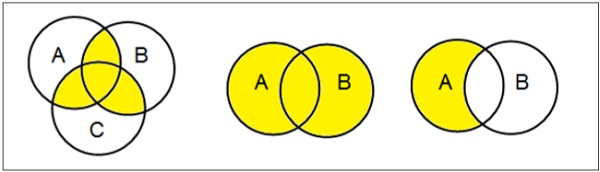## Set Operations

Set Operations include Set Union, Set Intersection, Set Difference, Complement of Set, and Cartesian Product.

### Set Union

The union of sets A and B (denoted by $A \cup B$) is the set of elements which are in A, in B, or in both A and B. Hence, $A \cup B = \lbrace x \:| \: x \in A\ OR\ x \in B \rbrace$.

Example − If $A = \lbrace 10, 11, 12, 13 \rbrace$ and B = $\lbrace 13, 14, 15 \rbrace$, then $A \cup B = \lbrace 10, 11, 12, 13, 14, 15 \rbrace$. (The common element occurs only once)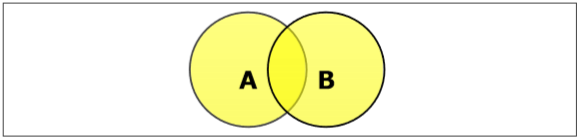### Set Intersection

The intersection of sets A and B (denoted by $A \cap B$) is the set of elements which are in both A and B. Hence, $A \cap B = \lbrace x \:|\: x \in A\ AND\ x \in B \rbrace$.

Example − If $A = \lbrace 11, 12, 13 \rbrace$ and $B = \lbrace 13, 14, 15 \rbrace$, then $A \cap B = \lbrace 13 \rbrace$.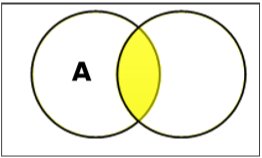### Set Difference/ Relative Complement

The set difference of sets A and B (denoted by $A – B$) is the set of elements which are only in A but not in B. Hence, $A - B = \lbrace x \:| \: x \in A\ AND\ x \notin B \rbrace$.

Example − If $A = \lbrace 10, 11, 12, 13 \rbrace$ and $B = \lbrace 13, 14, 15 \rbrace$, then $(A - B) = \lbrace 10, 11, 12 \rbrace$ and $(B - A) = \lbrace 14, 15 \rbrace$. Here, we can see $(A - B) \ne (B - A)$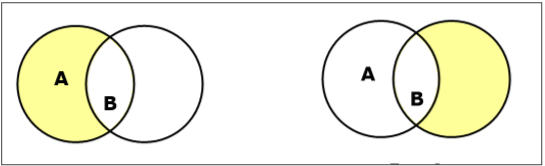### Complement of a Set

The complement of a set A (denoted by $A’$) is the set of elements which are not in set A. Hence, $A' = \lbrace x | x \notin A \rbrace$.

More specifically, $A'= (U - A)$ where $U$ is a universal set which contains all objects.

Example − If $A = \lbrace x \:| \: x\ \: {belongs\: to\: set\: of\: odd \:integers} \rbrace$ then $A' = \lbrace y\: | \: y\ \: {does\: not\: belong\: to\: set\: of\: odd\: integers } \rbrace$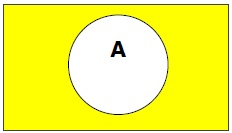### Cartesian Product / Cross Product

The Cartesian product of n number of sets $A_1, A_2, \dots A_n$ denoted as $A_1 \times A_2 \dots \times A_n$ can be defined as all possible ordered pairs $(x_1, x_2, \dots x_n)$ where $x_1 \in A_1, x_2 \in A_2, \dots x_n \in A_n$

Example − If we take two sets $A = \lbrace a, b \rbrace$ and $B = \lbrace 1, 2 \rbrace$,

The Cartesian product of A and B is written as − $A \times B = \lbrace (a, 1), (a, 2), (b, 1), (b, 2)\rbrace$

The Cartesian product of B and A is written as − $B \times A = \lbrace (1, a), (1, b), (2, a), (2, b)\rbrace$

## Power Set

Power set of a set S is the set of all subsets of S including the empty set. The cardinality of a power set of a set S of cardinality n is $2^n$. Power set is denoted as $P(S)$.

Example −

For a set $S = \lbrace a, b, c, d \rbrace$ let us calculate the subsets −

• Subsets with 0 elements − $\lbrace \emptyset \rbrace$ (the empty set)

• Subsets with 1 element − $\lbrace a \rbrace, \lbrace b \rbrace, \lbrace c \rbrace, \lbrace d \rbrace$

• Subsets with 2 elements − $\lbrace a, b \rbrace, \lbrace a,c \rbrace, \lbrace a, d \rbrace, \lbrace b, c \rbrace, \lbrace b,d \rbrace,\lbrace c,d \rbrace$

• Subsets with 3 elements − $\lbrace a ,b, c\rbrace,\lbrace a, b, d \rbrace, \lbrace a,c,d \rbrace,\lbrace b,c,d \rbrace$

• Subsets with 4 elements − $\lbrace a, b, c, d \rbrace$

Hence, $P(S)=$

$\lbrace \quad \lbrace \emptyset \rbrace, \lbrace a \rbrace, \lbrace b \rbrace, \lbrace c \rbrace, \lbrace d \rbrace, \lbrace a,b \rbrace, \lbrace a,c \rbrace, \lbrace a,d \rbrace, \lbrace b,c \rbrace, \lbrace b,d \rbrace, \lbrace c,d \rbrace, \lbrace a,b,c \rbrace, \lbrace a,b,d \rbrace, \lbrace a,c,d \rbrace, \lbrace b,c,d \rbrace, \lbrace a,b,c,d \rbrace \quad \rbrace$

$| P(S) | = 2^4 = 16$

Note − The power set of an empty set is also an empty set.

$| P (\lbrace \emptyset \rbrace) | = 2^0 = 1$

## Partitioning of a Set

Partition of a set, say S, is a collection of n disjoint subsets, say $P_1, P_2, \dots P_n$ that satisfies the following three conditions −

• $P_i$ does not contain the empty set.

$\lbrack P_i \ne \lbrace \emptyset \rbrace\ for\ all\ 0 \lt i \le n \rbrack$

• The union of the subsets must equal the entire original set.

$\lbrack P_1 \cup P_2 \cup \dots \cup P_n = S \rbrack$

• The intersection of any two distinct sets is empty.

$\lbrack P_a \cap P_b = \lbrace \emptyset \rbrace,\ for\ a \ne b\ where\ n \ge a,\: b \ge 0 \rbrack$

Example

Let $S = \lbrace a, b, c, d, e, f, g, h \rbrace$

One probable partitioning is $\lbrace a \rbrace, \lbrace b, c, d \rbrace, \lbrace e, f, g, h \rbrace$

Another probable partitioning is $\lbrace a, b \rbrace, \lbrace c, d \rbrace, \lbrace e, f, g, h \rbrace$

### Bell Numbers

Bell numbers give the count of the number of ways to partition a set. They are denoted by $B_n$ where n is the cardinality of the set.

Example

Let $S = \lbrace 1, 2, 3\rbrace$, $n = |S| = 3$

The alternate partitions are −

1. $\emptyset , \lbrace 1, 2, 3 \rbrace$

2. $\lbrace 1 \rbrace , \lbrace 2, 3 \rbrace$

3. $\lbrace 1, 2 \rbrace , \lbrace 3 \rbrace$

4. $\lbrace 1, 3 \rbrace , \lbrace 2 \rbrace$

5. $\lbrace 1 \rbrace , \lbrace 2 \rbrace , \lbrace 3 \rbrace$

Hence $B_3 = 5$